Main Restorations Software Audio/Jukebox/MP3 Everything Else Buy/Sell/Trade Project Announcements Monitor/Video GroovyMAME Merit/JVL Touchscreen Meet Up Retail Vendors Driving & Racing Woodworking Software Support Forums Consoles Project Arcade Reviews Automated Projects Artwork Frontend Support Forums Pinball Forum Discussion Old Boards Raspberry Pi & Dev Board controls.dat Linux Miscellaneous Arcade Wiki Discussion Old Archives Lightguns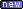Arcade1Up--- Bug Reports --- Site News Unread posts | New Replies | Recent posts | Rules | Chatroom | Wiki | File Repository | RSS | Submit news

###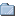Author Topic: Skin Question  (Read 2349 times)

0 Members and 1 Guest are viewing this topic.

#### Walkinshaw

• Trade Count: (0)
• Full Member
•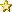•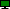Offline
•• Posts: 44
• Last login:April 14, 2009, 12:02:57 am
• I'm a llama!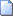##### Skin Question
« on: February 10, 2006, 01:01:47 am »
Hi guys,

I can't seem to find an answer to this.

I want to be able to have a skin that has numbers only as the selections as I would just like to run this off a number keypad. There is no need for letters.

Do I have to create a new background graphic with just numbers as apposed to Chris's Letter number combo. I want to use the BGCW skin but just have numbers as the selections.

Also what else would I need to do ?

Thanks

#### Chris

• Moderator
• Trade Count: (+2)
• Full Member
•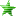•Offline
• Posts: 4575
• Last login:September 16, 2019, 04:28:11 pm##### Re: Skin Question
« Reply #1 on: February 10, 2006, 08:43:30 am »
Yes, you need to alter the backdrop and alter the skin file to reflect your keypad.
I'm not sure which skin you're using (I don't recall one called BGCW, but I'm at work and don't have the full package with me), but the setup should be the same for most of the skins....

Edit the .SKN file you are using with a text editor, and look for the Selection section.  For the default skin, it looks like this:

Code: [Select]
`[Selection]# SelectionMethod: Alphanumeric,Numeric,SingleLetter,SingleNumberSelectionMethod=AlphanumericUseZero=FalseHighLetter=DHighNumber=4`
(Hmm, I need to update this: SingleLetter and SingleNumber are out of date)

To set up your keypad, change the SelectionMethod to Numeric, the HighNumber to the highest number on your keypad, and UseZero to True or False depending on whether or not your keypad has a zero. You can ignore or remove the HighLetter setting.

Next, you will need to make sure all of the digits for your keypad are mapped to what you expect them to be in CONTROLS.INI.  By default, all of the keys on the numeric keypad are mapped to the corresponding buttons, but only digits 1 through 4 are mapped along the top of the keyboard.  If you are using a matrix-style keypad mapped through a keyboard encoder, your button assignments will be all over the map.

Finally, you need to figure out the new addresses of all of the selections so you can edit the graphic.  It's really not difficult to understand, but it's kinda hard to explain....

At some point, all of the selection addresses need to convert to an input value.  If you think of the default alphanumeric as a base-4 value with no zero, and the letters as the digits 1 through 4, you get the following address map:

A1=0
A2=1
A3=2
A4=3
B1=4
B2=5
B3=6
--BINGO! Either that, or I was attempting to say "before" but it was too many letters to type--=7
C1=8
C2=9
C3=10
C4=11
D1=12
D2=13
D3=14
D4=15

So with a numeric keypad, you simply use the base of the highest digit on your keypad, or thehighest digit plus 1 if you are including the zero.  Simple, huh?  Not really, there's an added complication.  If you are using the zero, yoy would think that the first selection would be 00, but since I don't believe any real jukebox would be set up that way, if you are using zeroes the first selection is always 01.

So if you are using a 10-key keypad with zeroes:

A1=0=01
A2=1=02
A3=2=03
A4=3=04
B1=4=05
B2=5=06
B3=6=07
--BINGO! Either that, or I was attempting to say "before" but it was too many letters to type--=7=08
C1=8=09
C2=9=10
C3=10=11
C4=11=12
D1=12=13
D2=13=14
D3=14=15
D4=15=16

If you are using, for example, a 6 digit keypad with no zero:

A1=0=11
A2=1=12
A3=2=13
A4=3=14
B1=4=15
B2=5=16
B3=6=21
--BINGO! Either that, or I was attempting to say "before" but it was too many letters to type--=7=22
C1=8=23
C2=9=24
C3=10=25
C4=11=26
D1=12=31
D2=13=32
D3=14=33
D4=15=34

The most efficient option is to use a 4-digit keypad with no zero:

A1=0=11
A2=1=12
A3=2=13
A4=3=14
B1=4=21
B2=5=22
B3=6=23
--BINGO! Either that, or I was attempting to say "before" but it was too many letters to type--=7=24
C1=8=31
C2=9=32
C3=10=33
C4=11=34
D1=12=41
D2=13=42
D3=14=43
D4=15=44

In fact, if you use 4 number buttons, you could cheat, label button 1 to A/1, 2 to B/2, etc., set the AlphaToggle option in JUKEBOX.INI to True, and leave the skin alone.

If none of this makes sense, let me know more about your exact set-up and I can be more specific.

--Chris
--Chris
DOSCab/WinCab Jukebox: http://www.dwjukebox.com

• Trade Count: (0)
• Full Member
••Offline
•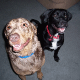• Posts: 34
• Last login:July 30, 2014, 12:03:09 pm
• Chip and Dale##### Re: Skin Question
« Reply #2 on: February 10, 2006, 05:40:48 pm »
I use a 100 selection numeric keypad skin.  Details below.

jukebox.ini:
[Display]
Skin = skins\newskin.skn
Width = 1024
Height = 768
Rotation = 0
FontSmoothing = True
FixedDimension = Auto
MonitorAspectRatio = 1.3333334
InsertCoinMsg1=No Selections Remaining
InsertCoinMsg2=Insert Coin
DisplayFont = jbdotmat.fnt
MousePointer = jbdefptr.ptr
AnimationSpeed = 30
[Settings]
DebugLevel = 0
RequireCredits = FALSE
PlayStimulatorDelay = 30
MaxRandomSonglength = 6
RandomSongPopularityPreference = 0
SongDelay = 2
MostPopularDisplayTime = 5
SongSort = Artist
KeyboardLEDsOff = False
ArtCacheMB = 4
MaxQueueSize = 500
AllowDuplicatesInQueue = True
SystemShutdownEnabled=False
[Controls]
Controls = controls.ini
KeyRepeatRate = 5
AutoSelect = True
AlphaToggle = False
Mouse = Enabled
[VolumeControl]
Volume = 100
SoundEffectsVolume=50
SoundEffectsVolumeWhenPlaying=30
VolumeIncrement = 10
SetSystemVolume = False
[Index]
SongPath1 = C:\My Documents\My Music
SongPath2 = D:\
UseID3 = True
UpdateIndex = TrueSmartCrop = True
MaxFolderDepth = 3DefaultAlbum = Singles
DefaultArtist = Various Artists
[ScreenSaver]
ScreenSaverTimeout = 5ScreenSaverCancelTimeout = 5
LineCount = 80
ColorVariance = 100
StepSize = 5
CornerMirror = True
ShowSongInfo = True

controls.ini:
[Controls]
KEY_ESC = BTN_1
KEY_ASTERISK = BTN_3
KEY_BACKSPACE = BTN_NEXTPG

Skin:
[skin]
background=blank.pcx
[Selection]
SelectionMethod=Numeric
UseZero=True
HighNumber=9
[Display1]
# Now Playing display
enabled=True
x=20
y=26
w=450
h=70
lines=4
color=turquoise
bgcolor=black
[Display2]
# Song List display
enabled=True
x=552
y=26
w=450
h=70
lines=4
color=turquoise
bgcolor=black
[Display3]
# Selection display
enabled=True
x=488
y=35
w=50
h=40
color=turquoise
bgcolor=black
[Display4]
# Credit Display
enabled=False
x=491
y=385
w=36
h=40
color=turquoise
bgcolor=Black
[TitleStrips]
Background=jb45lb04.jpg
#bgcolor=black
SongsPerStrip=Double
Font=bluehigh.ttf
FontSize=18
FontColor=Black
SmallFont=bluecond.ttf
SmallFontSize=18
Width=180
Height=60
StripCount=50
FontSmoothing=false
ForceUpperCaseTitles=False
ForceUpperCaseArtists=True
Animation=SlideHorizontal
[StripGrid]
x=20
y=110
rows=10
cols=5
rowspace=5
colspace=20
order=Horizontal
[Icon1]
x=161
y=2
w=130
h=20
OnImage=bj_pause.jpg
OffImage=bj_play.jpg
Clickable=False
Action=MSG_SONGPAUSE
[Icon2]
x=710
y=2
w=130
h=20
OnImage=bj_pop.jpg
OffImage=bj_cmgup.jpg
Clickable=False
Action=MSG_POPULAR
[Sounds]
BTN_0 = click2.wav
BTN_7 = click2.wav
BTN_8 = click2.wav
BTN_9 = click2.wav
BTN_4 = click2.wav
BTN_5 = click2.wav
BTN_6 = click2.wav
BTN_1 = click2.wav
BTN_2 = click2.wav
BTN_3 = click2.wav
I love Windows ME.  Blue is my favorite color.

#### Chris

• Moderator
• Trade Count: (+2)
• Full Member
••Offline
• Posts: 4575
• Last login:September 16, 2019, 04:28:11 pm##### Re: Skin Question
« Reply #3 on: February 10, 2006, 06:36:08 pm »
Can you actually select item 00 from the keypad that way?  Seems like the way I have it designed that shouldn't work...

--Chris
--Chris
DOSCab/WinCab Jukebox: http://www.dwjukebox.com

• Trade Count: (0)
• Full Member
••Offline
•• Posts: 34
• Last login:July 30, 2014, 12:03:09 pm
• Chip and Dale##### Re: Skin Question
« Reply #4 on: February 10, 2006, 09:44:53 pm »
Works like a charmI love Windows ME.  Blue is my favorite color.

#### Chris

• Moderator
• Trade Count: (+2)
• Full Member
••Offline
• Posts: 4575
• Last login:September 16, 2019, 04:28:11 pm##### Re: Skin Question
« Reply #5 on: February 11, 2006, 12:24:39 am »
Hmm... I probably only implemented that for CD skins, then.  I need to look at my code more often!
--Chris
DOSCab/WinCab Jukebox: http://www.dwjukebox.com